• 线性拟合是怎么实现的 线性拟合怎么优化 当时一时兴起的我就马上跑去百度谷歌了一番，发现似乎并没有想象的这么简单，于是先简单总结一下： 线性拟合是什么？ 线性拟合是曲线拟合的一种形式。设x和y都是被观测的量...
最近接到一个问题：

线性拟合是怎么实现的
线性拟合怎么优化

当时一时兴起的我就马上跑去百度谷歌了一番，发现似乎并没有想象的这么简单，于是先简单总结一下：
线性拟合是什么？

线性拟合是曲线拟合的一种形式。设x和y都是被观测的量，且y是x的函数：y=f(x; b)，曲线拟合就是通过x,y的观测值来寻求参数b的最佳估计值，及寻求最佳的理论曲线y=f(x; b)。当函数y=f(x; b)为关于b的i线性函数时，称这种曲线拟合为线性拟合。（摘自百度百科）

说白了，就是将一堆包含x，y值的坐标点放在一个二维平面，然后用尽可能接近每个点的一条曲线去描述它，鉴于问题太多，我这里就先讲讲直线的拟合叭
作为一组数据（x，y各一组应该是两组才对），应该有自己的想法，自己上二维数组的画板才对了呀（python可以用matplotlib.pyplot里的方法简易实现）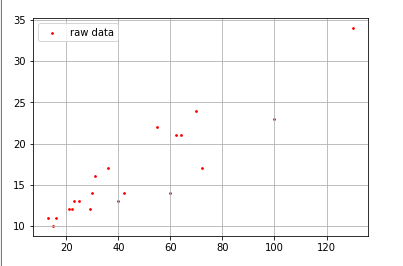好的那么它上去了，我们继续。
线性拟合怎么实现？
这是个好问题，我们想将数据拟合成一条直线，如果是用纸笔我们会怎么做呢
“哎，这么简单，不就是用尺子量到差不多然后画一笔就好了嘛”
“像这样是吧，那我要怎么判断这条线是不是拟合的线呢？”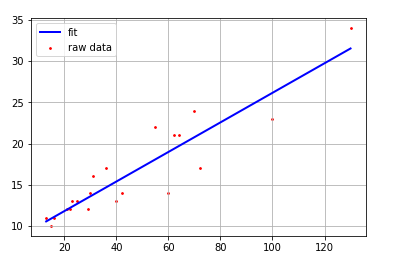“你看一哈子不就好咯”
好啦，言归正传，要将这个直线画出来呢，我们都知道一条直线可以用方程表述出来，也就是y = kx + b；其中呢，k 为直线的斜率，b 就是这条直线的 y 截距啦（没别的，就y = kx + b）
最小二乘法
作为一条直线，最重要的就是斜率和截距，作为一个耿直boy，那就直一点，直接将直线的斜率和截距求出来，这时候我们就用到了最小二乘法，而它是干嘛的呢：

最小二乘法（又称最小平方法）是一种数学优化技术。它通过最小化误差的平方和寻找数据的最佳函数匹配。利用最小二乘法可以简便地求得未知的数据，并使得这些求得的数据与实际数据之间误差的平方和为最小。最小二乘法还可用于曲线拟合。其他一些优化问题也可通过最小化能量或最大化熵用最小二乘法来表达。（摘自百度百科）

简单的说，就是求点到直线的垂直距离，根据横坐标来计算点到直线上垂直距离的差值。对这个点的值和拟合直线的距离求和，然后分别对k和b求导的方法来算出k和b的值。具体的求解过程可以看另一篇文章：

文章链接，还没有写，写后修改

距离图就如下啦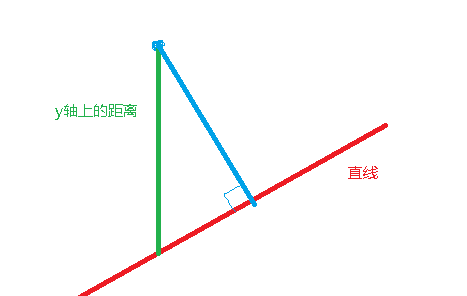#python代码如下：传入x和y两个数组，就会通过求平方和的方式得出结果，b,k的算法原理我会写在最小二乘法的原理上
def linefit(x,y):
N=int(len(x))
sumx,sumy,sumx2,sumxy=0,0,0,0
for i in range(0,N):
sumx+=x[i]
sumy+=y[i]
sumx2+=x[i]**2
sumxy+=x[i]*y[i]
b=(sumy*sumx2-sumx*sumxy)/(N*sumx2-sumx**2)
k=(N*sumxy-sumx*sumy)/(N*sumx2-sumx**2)
return b,k

梯度下降法
首先呢，我先来介绍一下梯度下降法：

如果有一个人想下山（没有很多鲁迅走过所成的路），那么怎样才会是下山最快的路呢？
首先，考虑一步能跨多远的距离，也就是步长
其次，我们要考虑向下走，那么我们就牵扯到向量了，将山的高度视为 y 轴，那么向下走最快的当然是走在一步以内能走到的距离内 y 减小的最多的那一步
然后我们每一步都这么考虑，直到到达最底下，于是我们的每一步都是下山相对减少最多的那一步
这样一次次迭代直到最后的极小值便是梯度下降法的结果，而过程则是梯度下降法

再来贴一贴百度百科的，是不是就能看懂了：

梯度下降法是一种迭代的思路求最优解，相对应的，我们在计算时，也要收集点到直线的距离，但是与最小二乘法不同的是，我们不需要通过计算直角三角形来得出斜率，因为在每一次迭代的时候我们就可以得出一条拟合的直线，迭代时的拟合直线为 y = ki x +bi。
此时提一下损失函数，也就是说，每一个点都不一定完全符合这个拟合的直线，肯定会有差值，这个差值就记为一个名为”损失“的值里，损失函数 Q 就是由每一个点到直线的距离的平方加起来的（是不是有点像最小二乘法，也就是Q = $\sum_{i=1}^m$(yi - (ki x + bi) )2 ）
那么我们要在何时停止呢，就可以设定一个停止的值，当再往下迭代，其损失函数的变化比约定的值小时，即判为梯度下降收敛了，即往下运算的性价比不高了，便可以退出
以下便是大致迭代过程
	epsilon = 0.01  #迭代伐值

alpha = 0.001 #迭代速率
theta0 = 0 #截距
theta1 = 0 #斜率

error1 = 0 #当前迭代的损失
error0 = 0 #上一次迭代的损失
while True:
sum0 = 0
sum1 = 0
cnt = cnt + 1
for i in range(0, len(x)):
#计算是线高了还是低了，低了会变成负值
diff =y[i] - (theta0 + theta1 * x[i])

sum0 = sum0 + diff
sum1 = sum1 + diff * x[i]

theta0 = theta0 + alpha * sum0 / len(x)#在这次迭代的变化
theta1 = theta1 + alpha * sum1 / len(x)#在这次迭代的变化

error1 = 0 #重置此次迭代的损失
for i in range(0, len(x)):
error1 = error1 + (y[i] - (theta0 + theta1 * x[i] )) ** 2 #计算损失

if abs(error1 - error0) < epsilon:#损失小于约定值
break
else:
error0 = error1

线性拟合的优化
线性拟合的思路我们讲清楚了，那么我们该怎么优化呢？
首先，我们可以分为两个部分：

1：对数据进行筛选
2.：对函数进行优化
3：对函数的结果进行优化

筛选数据
想要使直线尽可能拟合成一个更正确的直线，我们可以舍弃掉一部分特别偏的点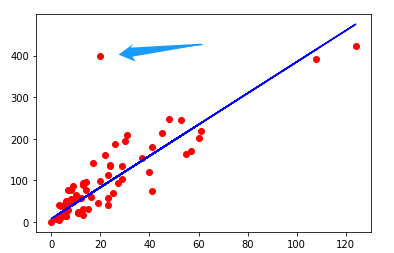比如这里就出现了一个跟直线打着八竿子远的点，当然，这么远的点对我们的直线拟合的速度肯定是有影响的，我们就应该将这个数据排除在外，筛选出一定范围内拟合直线的点，可以提高对数据的运算速度。
那么我们该怎么筛选出这些点呢？
首先介绍最常用的一种方式：标准偏差筛选法
我们都知道，数据筛选是将相对较远的无用的数据给筛出，那么怎样的距离才算较远呢？如果自定一个常数那么也不能适应所有数据情况，所以这个值我们应该从数据中得出，于是我们想到了标准偏差。
标准偏差很好求，也就是平均方差开方，而平均方差也就是方差和然后除以样本数量求平均值
Avg = $\frac{1}{m}$$\sum_{i=1}^m$(xi-$\overline{x}$)2
然后呢，我们以三倍标准方差为阈值，当一个点的标准差大于三倍标准偏差，那么我们就可以认定它为无用值，将其排除。
这种做法的好处就是将一些太偏的数据给除掉，在梯度下降中可以使迭代次数减少
函数优化
以梯度下降为例，之前提到过，我们梯度下降法可以看成是一步一步下山的方法，那么我们该选取怎样的步长则是优化点之一，太长了，我们一不小心就从这座山跨过去了跨到另一座山上；太短了，又要走很多次，导致结果变慢（如图就太长了要反复横跳）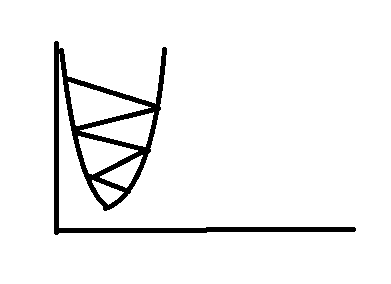所以说确定步长也是算法中的一个优化点，当然也有公式可以求得最优步长（详情可见armijo算法，太复杂不解释）
然后第二个就是减少参与运算的数据
首先我来介绍三种梯度下降的算法：
1.批量梯度下降法
2.随机梯度下降法
3.小批量梯度下降法
首先是批量梯度下降法，也就是之前的使用所有数据来进行更新，比如我有m个样本，就将所有m个样本用于更新算法，因为是用所有样本，所以当数据量特别大的时候运算速度还是很慢的
第二个就是随机梯度下降法，与批量梯度下降法是两个极端，为了改变批量梯度下降法的缺点，我们每次迭代时都只随机选取一个作为样本，迭代速度就快很多，也就是有m个样本，但是每次更新只选用1个，优点是运算较快，但缺点也显而易见，就是收敛的时候梯度方向变化很大，不容易达到收敛的局部最优解
第三个就是小批量梯度下降法，吸收了批量梯度和随机梯度的优点，我们从m个样本中选若干个（一般选10个）作为样本进行迭代，相比于大量的m来说，小批量的10个运算速度还是非常快的，相比于收敛梯度方向不稳定的随机梯度下降法来说10个样本同时运算的收敛方向变化不算太大，结合了两者的优点
函数结果优化
基于梯度下降来说，梯度下降法找的是损失函数最小的那个值，但是如果所寻找的局部最优值不是全局最优值呢？那么我们最后得到的结果就不一定是最满意的结果，所以我们本应该继续迭代寻找全局最优值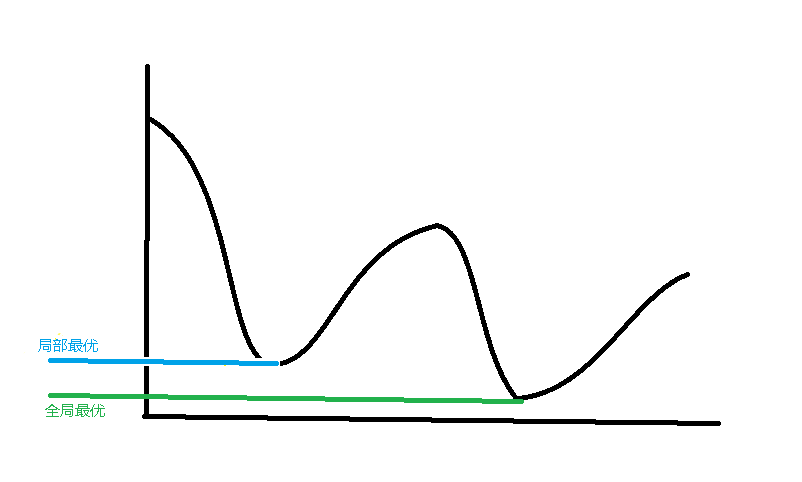那咋办呢，看到这个坑就想往里跳，跳了还懒得出去。这时候我们就要催一催这个函数继续往前探索一段路，如果在前边找到了比局部最优还好的解，那就替换掉，如果在一定路程还没找到的，那就回去吧。
于是，模拟退火算法就出现啦。

模拟退火算法从某一较高初温出发，伴随温度参数的不断下降,结合概率突跳特性在解空间中随机寻找目标函数的全局最优解，即在局部最优解能概率性地跳出并最终趋于全局最优。（摘自百度百科）

简单地说，在打铁退火就像是往前探索，横坐标即是温度，纵坐标便是铁的柔软程度，在打铁的时候，随着温度下降，铁的柔软程度会逐渐变化，而我们要做的就是在温度区间内找到最坚硬的部分（值最低）
而在解决问题时，横坐标即是问题的迭代次数，纵坐标即是所求的最小值（损失函数最小值），我们要求的就是在规定迭代区间内找出其最小值。

模拟退火的基本思想:
(1) 初始化：初始温度T(充分大)，初始解状态S(是算法迭代的起点)，每个T值的迭代次数L
(2) 对k=1, …, L做第(3)至第6步：
(3) 产生新解S′
(4) 计算增量ΔT=C(S′)-C(S)，其中C(S)为评价函数
(5) 若ΔT<0则接受S′作为新的当前解，否则以概率exp(-ΔT/T)接受S′作为新的当前解.
(6) 如果满足终止条件则输出当前解作为最优解，结束程序。
终止条件通常取为连续若干个新解都没有被接受时终止算法。
(7) T逐渐减少，且T->0，然后转第2步。

然后我再来贴一下“人话”

模拟退火算法:
退火算法是一种贪心算法,但是引入了随机因素,以一种随机概率接收比当前解要差的结果
其中,概率可以根据当前温度以及解的最优程度决定:
概率P、能量差dE、温度T、自然对数e dE = Enew - Eold  //新的值减去旧的值
其中dE的值分两种情况：
若dE<0,也就是新的能量值小于旧的能量值，也就是得到趋向于最小值的一个值时，则必定接受 此时的P(dE)将直接为1
若dE>0，也就是新的能量值大于旧的能量值，此时就要考虑是否将这个值采纳，则使用公式：
P(dE) = e(-(dE/T))
其中:T为迭代时的温度,随着迭代次数的增加,T的值将会减小(也就是退火的过程,温度逐渐降低)
随着T降低,dE/T会增大,所以e(-(dE/T))将会趋近于0,所以温度越低,被接受的概率也会随之降低
于是在循环中,我们只需要将温度设置为循环结束条件即可,每次循环将温度递减

/*
T:当前温度
T_min:温度下限
newState:新的状态
oldState:旧的状态
nextState:循环的下一个状态
dE:两个状态的能量差
P(dE):接收新状态的概率
getLow:接受新状态的最低接收值
r:温度递减系数
*/

void SA()
{
while(T > T_min)
{
dE = E( newState ) - E( oldState );
if(dE >= 0)
{
oldState = newState;
}
else
{
if( P(dE) > getLow)
{
oldState = newState;
}
}
T = r * T;
nextState;
}
}

在线性拟合中呢，我们需要找的最小值就是在梯度下降法中有提到过的损失函数Q，当Q最小时，也就是所有点到直线距离都为最短，那么这条直线就是我们要求的直线。
在模拟退火算法中呢，有关键的点，就是初始温度T、结束温度T_min和接受的概率P（dE），其中呢P（dE）是根据温度和前后两种情况的差值决定的，温度越低，取值的概率越低。


展开全文算法
• 曲线拟合(Curve Fitting)的数学定义是指用连续曲线近似地刻画或比拟平面上一离散点所表示的坐标之间的函数关系，是一种用解析表达式逼近离散数据的方法。曲线拟合通俗的说法就是“拉曲线”，也就是将现有数据透过...
12.1 曲线拟合

12.1.1 曲线拟合的定义

曲线拟合(Curve Fitting)的数学定义是指用连续曲线近似地刻画或比拟平面上一组离散点所表示的坐标之间的函数关系，是一种用解析表达式逼近离散数据的方法。曲线拟合通俗的说法就是“拉曲线”，也就是将现有数据透过数学方法来代入一条数学方程式的表示方法。科学和工程遇到的很多问题，往往只能通过诸如采样、实验等方法获得若干离散的数据，根据这些数据，如果能够找到一个连续的函数（也就是曲线）或者更加密集的离散方程，使得实验数据与方程的曲线能够在最大程度上近似吻合，就可以根据曲线方程对数据进行数学计算，对实验结果进行理论分析，甚至对某些不具备测量条件的位置的结果进行估算。

12.1.2 简单线性数据拟合的例子

回想一下中学物理课的“速度与加速度”实验：假设某物体正在做加速运动，加速度未知，某实验人员从时间t0 = 3秒时刻开始，以1秒时间间隔对这个物体连续进行了12次测速，得到一组速度和时间的离散数据，请根据实验结果推算该物体的加速度。

时间 (秒)

3

4

5

6

7

8

9

10

速度（米/秒）

8.41

9.94

11.58

13.02

14.33

15.92

17.54

19.22

时间 (秒)

11

12

13

14

速度（米/秒）

20.49

22.01

23.53

24.47

表 12 – 1 物体速度和时间的测量关系表

在选择了合适的坐标刻度之后，我们就可以在坐标纸上画出这些点。如图12–1所示，排除偏差明显偏大的测量值后，可以看出测量结果呈现典型的线性特征。沿着该线性特征画一条直线，使尽量多的测量点能够位于直线上，或与直线的偏差尽量小，这条直线就是我们根据测量结果拟合的速度与时间的函数关系。最后在坐标纸上测量出直线的斜率K，K就是被测物体的加速度，经过测量，我们实验测到的物体加速度值是1.48米/秒2。

图 12 – 1 实验法测量加速度的过程

12.2 最小二乘法曲线拟合

使用数学分析进行曲线拟合有很多常用的方法，这一节我们先介绍一下最简单的最小二乘法，并给出使用最小二乘法解决上一节给出的速度与加速度实验问题。

12.2.1 最小二乘法原理

下面以多项式模式为例，介绍一下使用最小二乘法进行曲线拟合的完整步骤。假设选择的拟合多项式模式是：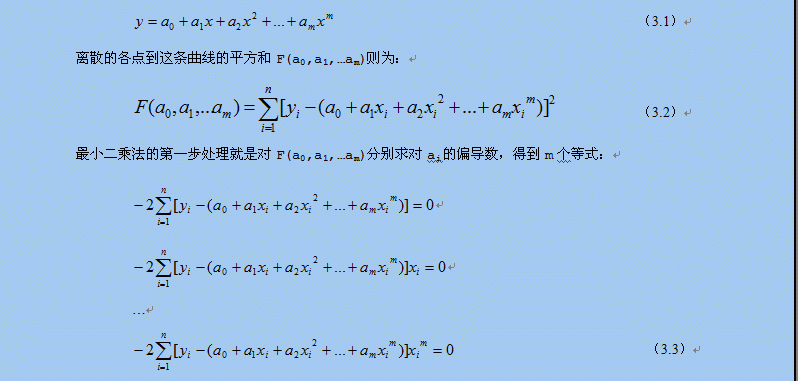这m个等式相当于m个方程，a0,a1,…am是m个未知量，因此这m个方程组成的方程组是可解的，最小二乘法的第二步处理就是将其整理为针对a0,a1,…am的正规方程组。最终整理的方程组如下：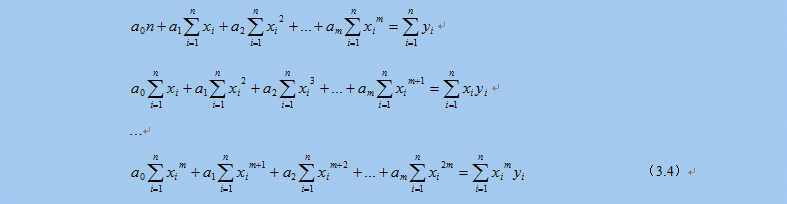最小二乘法的第三步处理就是求解这个多元一次方程组，得到多项式的系数a0,a1,…am，，就可以得到曲线的拟合多项式函数。求解多元一次方程组的方法很多，高斯消元法是最常用的一种方法，下一节就简单介绍一下最小二乘算法实现所用的高斯消元法算法。

12.2.2 高斯消元法求解方程组

在数学上，高斯消元法是线性代数中的一个算法，可用来求解多元一次线性方程组，也可以用来求矩阵的秩，以及求可逆方阵的逆矩阵。高斯消元法虽然以数学家高斯的名字命名，但是最早出现在文献资料中应该是中国的《九章算术》。

高斯消元法的主要思想是通过对系数矩阵进行行变换，将方程组的系数矩阵由对称矩阵变为三角矩阵，从而达到消元的目的，最后通过回代逐个获得方程组的解。在消元的过程中，如果某一行的对角线元素的值太小，在计算过程中就会出现很大的数除以很小的数的情况，有除法溢出的可能，因此在消元的过程中，通常都会增加一个主元选择的步骤，通过行交换操作，将当前列绝对值最大的行交换到当前行位置，避免了除法溢出问题，增加了算法的稳定性。

高斯消元法算法实现简单，主要有两个步骤组成，第一个步骤就是通过选择主元，逐行消元，最终行程方程组系数矩阵的三角矩阵形式，第二个步骤就是逐步回代的过程，最终矩阵的对角线上的元素就是方程组的解。下面就给出高斯消元法的一个算法实现：

76 /*带列主元的高斯消去法解方程组，最后的解在matrixA的对角线上*/

77 bool GuassEquation::Resolve(std::vector<double>& xValue)

78 {

79     assert(xValue.size() == m_DIM);

80

81     /*消元，得到上三角阵*/

82     for(int i = 0; i < m_DIM - 1; i++)

83     {

84         /*按列选主元*/

85         int pivotRow = SelectPivotalElement(i);

86         if(pivotRow != i)/*如果有必要，交换行*/

87         {

88             SwapRow(i, pivotRow);

89         }

90         if(IsPrecisionZero(m_matrixA[i * m_DIM + i])) /*主元是0? 不存在唯一解*/

91         {

92             return false;

93         }

94         /*对系数归一化处理，使行第一个系数是1.0*/

95         SimplePivotalRow(i, i);

96         /*逐行进行消元*/

97         for(int j = i + 1; j < m_DIM; j++)

98         {

99             RowElimination(i, j, i);

100         }

101     }

102     /*回代求解*/

103     m_matrixA[(m_DIM - 1) * m_DIM + m_DIM - 1] = m_bVal[m_DIM - 1] / m_matrixA[(m_DIM - 1) * m_DIM + m_DIM - 1];

104     for(int i = m_DIM - 2; i >= 0; i--)

105     {

106         double totalCof = 0.0;

107         for(int j = i + 1; j < m_DIM; j++)

108         {

109             totalCof += m_matrixA[i * m_DIM + j] * m_matrixA[j * m_DIM + j];

110         }

111         m_matrixA[i * m_DIM + i] = (m_bVal[i] - totalCof) / m_matrixA[i * m_DIM + i];

112     }

113

114     /*将对角线元素的解逐个存入解向量*/

115     for(int i = 0; i < m_DIM; i++)

116     {

117         xValue[i] = m_matrixA[i * m_DIM + i];

118     }

119

120     return true;

121 }

GuassEquation::Resolve()函数中m_matrixA是以一维数组形式存放的系数矩阵，m_DIM是矩阵的维数，SelectPivotalElement()函数从系数矩阵的第i列中选择绝对值最大的那个值所在的行，并返回行号，SwapRow()函数负责交换系数矩阵两个行的所有值，SimplePivotalRow()函数是归一化处理函数，通过除法操作将指定的行的对角线元素变换为1.0，以便简化随后的消元操作。

12.2.3 最小二乘法解决“速度与加速度”实验

根据12.2.1节对最小二乘法原理的分析，用程序实现最小二乘法曲线拟合的算法主要由两个步骤组成，第一个步骤就是根据给出的测量值生成关于拟合多项式系数的方程组，第二个步骤就是解这个方程组，求出拟合多项式的各个系数。根据对上文最终整理的正规方程组的分析，可以看出其系数有一定的关系，就是每一个方程式都比前一个方程式多乘了一个xi。因此，只需要完整计算出第一个方程式的系数，其他方程式的系数只是将前一个方程式的系数依次左移一位，然后单独计算出最后一个系数就可以了，此方法可以减少很多无谓的计算。求解多元一次方程组的方法就使用12.2.2节介绍的高斯消元法，其算法上一节已经给出。

这里给出一个最小二乘算法的完整实现，以12.1.2节的数据为例，因为数据结果明显呈现线性方程的特征，因此选择拟合多项式为v = v0 + at，v0和a就是要求解的拟合多项式系数。

99 bool LeastSquare(const std::vector<double>& x_value, const std::vector<double>& y_value,

100                  int M, std::vector<double>& a_value)

101 {

102     assert(x_value.size() == y_value.size());

103     assert(a_value.size() == M);

104

105     double *matrix = new double[M * M];

106     double *b= new double[M];

107

108     std::vector<double> x_m(x_value.size(), 1.0);

109     std::vector<double> y_i(y_value.size(), 0.0);

110     for(int i = 0; i < M; i++)

111     {

112         matrix[ARR_INDEX(0, i, M)] = std::accumulate(x_m.begin(), x_m.end(), 0.0);

113         for(int j = 0; j < static_cast<int>(y_value.size()); j++)

114         {

115             y_i[j] = x_m[j] * y_value[j];

116         }

117         b[i] = std::accumulate(y_i.begin(), y_i.end(), 0.0);

118         for(int k = 0; k < static_cast<int>(x_m.size()); k++)

119         {

120             x_m[k] *= x_value[k];

121         }

122     }

123     for(int row = 1; row < M; row++)

124     {

125         for(int i = 0; i < M - 1; i++)

126         {

127             matrix[ARR_INDEX(row, i, M)] = matrix[ARR_INDEX(row - 1, i + 1, M)];

128         }

129         matrix[ARR_INDEX(row, M - 1, M)] = std::accumulate(x_m.begin(), x_m.end(), 0.0);

130         for(int k = 0; k < static_cast<int>(x_m.size()); k++)

131         {

132             x_m[k] *= x_value[k];

133         }

134     }

135

136     GuassEquation equation(M, matrix, b);

137     delete[] matrix;

138     delete[] b;

139

140     return equation.Resolve(a_value);

141 }

将表12－1的数据带入算法，计算得到v0 = 4.05545455，a = 1.48818182，比作图法得到的结果更精确。以上算法是根据最小二乘法的理论推导系数方程，并求解系数方程得到拟合多项式的系数的一种实现方法，除此之外，还可以利用预先计算好的最小二乘解析理论直接求得拟合多项式的系数，读者可自行学习相关的实现算法。

12.3 三次样条曲线拟合

样条插值是一种工业设计中常用的、得到平滑曲线的一种插值方法，三次样条又是其中用的较为广泛的一种。使用三次样条曲线进行曲线拟合可以得到非常高精度的拟合结果，并且很容易得到拟合函数，本节的内容将重点介绍三次样条曲线拟合的原理和算法实现，并通过一个具体的例子将三次样条函数拟合的曲线与原始曲线对比显示，体会一下三次样条曲线拟合的惊人效果。

12.3.1 插值函数

前文提到过，曲线拟合的实质就是各种插值计算，因此，插值函数的选择决定了曲线拟合的效果。那么插值函数的数学定义是什么呢？若在[a, b]上给出n + 1个点a ≤ x0 < x1 < ⋯ < xn ≤ b，f(x) 是[a, b]上的实值函数, 要求一个具有n + 1个参量的函数s(x; a0,...,an) 使它满足

s(xi; a0,..., an) = f(xi) , i = 0, 1, ⋯, n                          （3.5）

则称s(x)为f(x) 在[a, b]上的插值函数. 若s(x) 关于参量a0, a1,...,an是线性关系, 即：

s(x) = a0s0(x) + a1s1(x) + ⋯ + ansn(x)                                （3.6）

s(x)就是多项式插值函数，如果si(x)是三角函数，则s(x)就是三角插值函数。

比较常用的多项式插值函数是牛顿插值多项式和拉格朗日插值多项式，但是在多项式的次数比较高的情况下，插值点数n过多会导致多项式插值在收敛性和稳定性上失去保证，因此，当插值点数n较大的情况下，一般不使用多项式插值，而采用样条插值或次数较低的最小二乘法插值。

12.3.2 样条函数的定义

在所有能够保证收敛性和稳定性的插值函数中，最常用的，也是最重要的插值函数就是样条插值函数。采用样条函数计算出的插值曲线和曲面在飞机、轮船和汽车等精密机械设计中都得到了广泛的应用。样条插值函数的数学定义是这样的：

设区间[a, b]上选取n - 1个节点（包括区间端点a和b共n + 1个节点），将其划分为n个子区间 a = x0 < x1 < ⋯ < xn = b, 如果存在函数s(x)，使得s(x)满足以下两个条件：

(1) s(x) 在整个区间[a, b]上具有m - 1阶连续导数；

(2) s(x)在每个子区间[xi-1, xi], i= 1, 2, ⋯, n 上是m 次代数多项式（最高次数为m次）；

则称s(x)是区间[a, b]上的m 次样条函数。假如区间[a, b]上存在实值函数f(x)，使得每个节点处的值f(xi)与s(xi)相等，即

s(xi) = f(xi), i = 0, 1, …, n                                          （3.7）

则称s(x)是实值函数f(x)的m 次样条插值函数。

当m = 1时，样条插值函数就是分段线性插值, 此时虽然s(x)是属于区间[a, b]上的函数, 但它不光滑（连一阶连续导数性质都不具备）,不能满足工程设计要求。工程设计通常使用较多的是m = 3时的三次样条插值函数，此时样条函数具有二阶连续导数性质。

根据三次样条函数的定义，s(x)在每个子区间上的样条函数si(x)都是一个三次多项式，也就是说，三次样条函数s(x)由n个区间上的n个三次多项式组成，每个三次多项式可描述为以下形式：

si(x) = aix3 + bix2 + cix + di      i = 1, 2, …, n                      （3.8）

因此，要确定完整的样条函数s(x)需要确定ai、bi、ci和di公4n个系数。根据样条函数的定义，s(x)在区间内的n - 1个节点处都是连续的，并且其一阶导数si‘(x)和二阶导数si“(x)都是连续的，根据连续函数的性质（xi的左右导数相等），我们可以得到3(n - 1)个条件：

si(xi - 0) = si+1(xi + 0)    i = 1, 2, …, n-1

si‘(xi - 0) = si+1‘(xi + 0)    i = 1, 2, …, n-1

si“(xi - 0) = si+1“(xi + 0)    i = 1, 2, …, n-1                          （3.9）

再加上插值函数在包括区间端点a（就是x0），b（就是xn）在内的n + 1个节点处满足s(xi) = f(xi)，又可以得到n + 1个条件，这样就具备了4n – 2个条件。

12.3.3 边界条件

为了解决4n个系数组成的方程组，最终确定的s(x)，需要再补充两个边界条件使之满足4n个条件。常用的边界条件有以下几种：

第一类边界条件，即满足s‘(x0) = f’(x0)，s‘(xn) = f’(xn)两个条件，其中f(x)是实值函数。

第二类边界条件，即满足s”(x0) = f”(x0)，s”(xn) = f”(xn)两个条件，其中f(x)是实值函数。特别情况下，当f”(x0) = f”(xn) = 0的时候，也就是s”(x0) = s”(xn) = 0的情况下，第二类边界条件又被称为自然边界条件。

当样条函数的实值函数f(x)是以[a, b]为周期的周期函数时，三次样条函数s(x)在两个端点处满足s‘(x0 - 0) = s‘(xn + 0)和s”(x0 - 0) = s”(xn + 0)，这种情况又被成为第三类边界条件。

工程技术中常用的是第一类边界条件和第二类边界条件，以及第二类边界条件的特殊情况自然边界条件。理想情况下，也就是实值函数已知的情况下，可以通过实值函数直接计算出边界条件的值，否则的话，就只能通过测量和计算得到边界条件的值，有时候甚至只能给出经验估计值，工程技术中通常根据实际情况灵活使用各类边界条件。

12.3.4 推导三次样条函数

求三次样条插值函数s(x)的方法很多，其基本原理都是首先求出由待定系数组成的s(x)，以及其一阶导数s’(x)和二阶导数s”(x)，然后将其带入到12.3.2和12.3.3节列举的4n个条件中，得到关于待定系数的方程组，最后求解方程组得到待定系数，并最终确定插值函数s(x)。

求三次样条插值函数s(x)常用的方法是“三转角法”和“三弯矩法”。根据三次样条函数的性质，s(x)的一阶导数s’(x)是二次多项式， 二阶导数s”(x)是一次多项式（线性函数）， “三转角法”和“三弯矩法”的主要区别是利用这两个特性推导插值函数s(x)、s’(x)和s”(x)的方式不同。“三转角法”利用s(x)的一阶导数s’(x)是二次多项式这个特性，对于子区间[xi, xi+1]，利用抛物线插值公式获得一个通过xi和xi+1两个点的二次多项式做为s’(x)，然后对s’(x)进行积分和微分（求导）运算，分别得到s(x)，和s”(x)，最后将它们带入4n个条件中求解系数方程组。“三弯矩法”则是利用s(x)的二阶导数s”(x)是一次多项式（线性函数）这个特性，对于子区间[xi, xi+1]，首先假设一个通过xi和xi+1两个点的线性函数做为s”(x)，然后对s”(x)进行连续两次积分运算得到s(x)，再对s(x)进行求导得运算到s’(x)，最后将它们带入4n个条件中求解系数方程组。这两种方法的本质是一样的，只是对s(x)的推导过程不同，接下来就介绍使用“三弯矩法”求解三次样条函数的方法。

三次样条函数的求解过程就是系数方程组的推导过程，使用“三弯矩法”推导系数方程组，首先要确定插值函数的二阶导数s”(x)。根据三次样条函数的性质，在每个子区间[xi, xi+1]上，其二阶导数s”(x)是个线性方程，现在假设在xi和xi+1两个端点的二阶导数值分别是Mi和Mi+1，也就是s”(xi) = Mi，s”(xi+1) = Mi+1，则经过xi和xi+1的两点式直线方程是：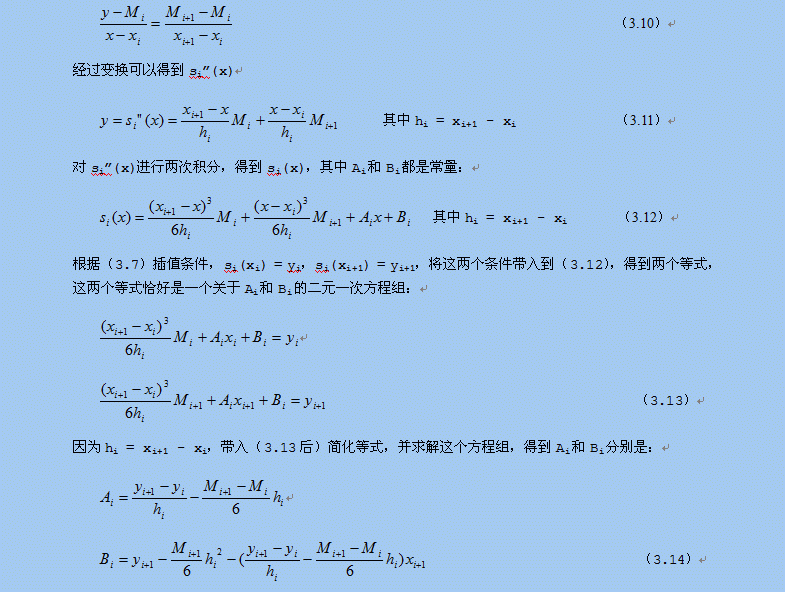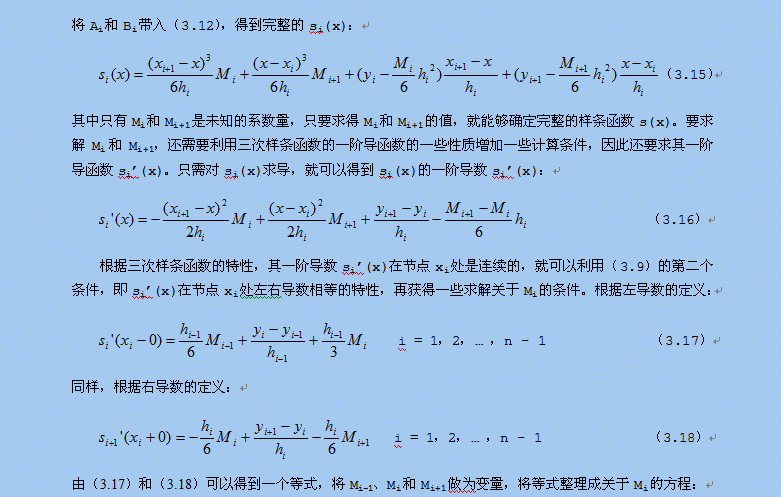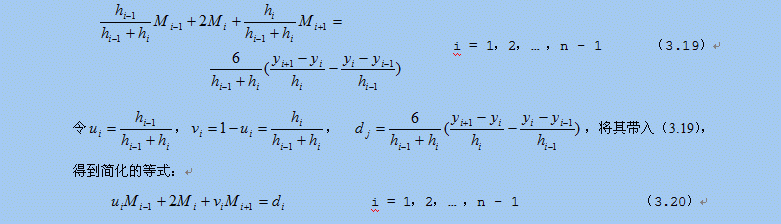从M0到Mn，有n+1个Mi的值需要求解，但是（3.20）只有n-1个等式，此时就需要用到两个边界条件了。

如果是使用第二类边界条件，则直接可以得到以下两个条件等式：

s”(x0) = M0 = f”(x0) = y0’                                                (3.21)

s”(xn) = Mn = f”(xn) = yn’                                                (3.22)

令d0 = 2y0’，dn = 2yn’，可以得到由第二类边界条件确定的两个方程：

2M0 = d0                                                                    (3.23)

2Mn = dn                                                                    (3.24)

如果是使用第一类边界条件，即s‘(x0) = f’(x0)，s‘(xn) = f’(xn)两个条件，则需要将这两个条件代入（3.16），通过计算得到两个条件等式。将s‘(x0) = y0’代入（3.16），得到：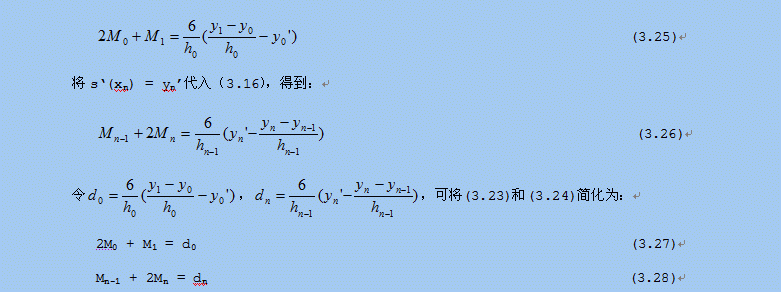将第二类边界条件得到的(3.23)和（3.24）或第一类边界条件得到的（3.27）和（3.28）与（3.20）中的n – 1个等式组合在一起就得到一个关于Mi的方程组，求解此方程组可以得到Mi的值，代入到（3.15）即可得到三次样条函数方程。以第一类边界条件得到的（3.27）和（3.28）为例，与（3.20）连立得到以下方程组：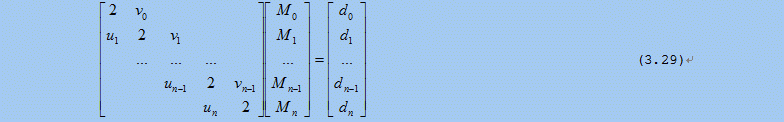这就是三弯矩方程组，其中Mi，i=0,1,…n就是三次样条函数s(x)的矩。根据（3.27）和（3.28），un = 1，v0 = 1，其余各系数可以通过（3.19）中的系数计算出来。这个方程组的系数矩阵是一个对角线矩阵，并且是一个严格对角占优的对角阵（ui和vi的值均小于主对角线的值，也就是ui和vi的值皆小于2），可以使用追赶法求解。下一节将介绍如何使用追赶法求解方程组，并给出求解的算法实现。

12.3.5 追赶法求解方程组

任意矩阵A，都可以通过克洛脱(Crout)分解得到两个三角矩阵：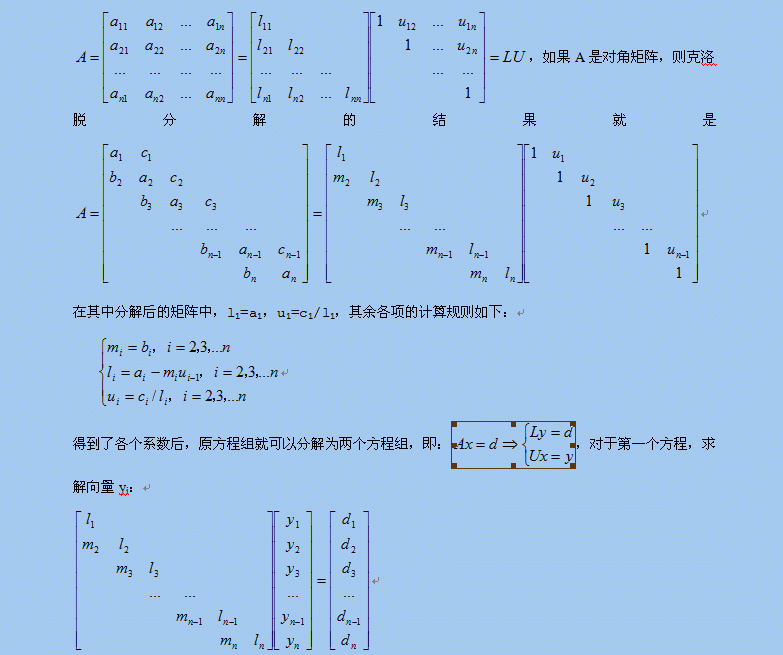其中y1=d1/l1，其余各项的递推计算关系是：

yi = (di – miyi-1)/li， i = 2，3，… ，n

对于第二个方程，求解最终结果xi：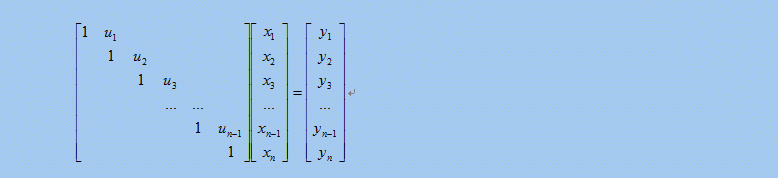其中xn = yn，其余各项的递推求解关系是：

xi = yi – uixi+1， i = n – 1, n – 2, …, 1

递推计算yi和xi的过程分别被形象地形容为追的过程和赶的过程，这也是追赶法得名的原因，实际上这种方法在国际上叫做托马斯（Thomas）法。在这里需要强调一下，对三角矩阵的克洛脱分解需要满足几个条件，否则无法进行，这几个条件分别是：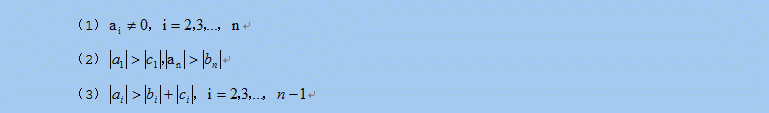下面就给出一个追赶法求解方程组的通用算法实现，在使用之前需要判断系数矩阵是否是对三角矩阵，并且满足上述三个条件，相关的判断请读者自行添加：

76 /*追赶法求对三角矩阵方程组的解*/

77 bool ThomasEquation::Resolve(std::vector<double>& xValue)

78 {

79     assert(xValue.size() == m_DIM);

80

81     std::vector<double> L(m_DIM);

82     std::vector<double> M(m_DIM);

83     std::vector<double> U(m_DIM);

84     std::vector<double> Y(m_DIM);

85

86     /*消元，追的过程*/

87     L = m_matrixA[ARR_INDEX(0, 0, m_DIM)];

88     U = m_matrixA[ARR_INDEX(0, 1, m_DIM)] / L;

89     Y = m_bVal / L;

90     for(int i = 1; i < m_DIM; i++)

91     {

92         if(IsPrecisionZero(m_matrixA[ARR_INDEX(i, i, m_DIM)]))

93         {

94             return false;

95         }

96         M[i] = m_matrixA[ARR_INDEX(i, i - 1, m_DIM)];

97         L[i] = m_matrixA[ARR_INDEX(i, i, m_DIM)] - M[i] * U[i - 1];

98         Y[i] = (m_bVal[i] - M[i] * Y[i - 1]) / L[i];

99         if((i + 1) < m_DIM)

100         {

101             U[i] = m_matrixA[ARR_INDEX(i, i + 1, m_DIM)] / L[i];

102         }

103     }

104     /*回代求解，赶的过程*/

105     xValue[m_DIM - 1] = Y[m_DIM - 1];

106     for(int i = m_DIM - 2; i >= 0; i--)

107     {

108         xValue[i] = Y[i] - U[i] * xValue[i + 1];

109     }

110

111     return true;

112 }

12.3.6 三次样条曲线拟合算法实现

根据12.3.4节对三次样条函数的推导分析，三次样条曲线拟合算法的核心可分为三部分，第一部分是根据推导结果计算关于三次样条函数的“矩”的方程组的系数矩阵，第二部分就是用追赶法求解方程组，得到各个区间的三次样条函数，第三部分就是根据每个拟合点的输入的值xi，确定使用哪个区间的三次样条函数，并使用该区间的三次样条函数计算出三次样条插值yi，最后得到的一系列（xi, yi）组成的曲线就是三次样条拟合曲线。拟合算法也是按照上面的分析，分三个步骤计算插值：

第一步是计算系数矩阵，其中u0、v0、d0和dn的值需要单独计算，其余的值可以通过（3.19）递推计算出来。

第二步是将系数矩阵代入12.3.5节给出的追赶法通用算法，求出Mi的值。求解之前，先证明一下第一步得到系数矩阵是否满足追赶法的条件。首先，主对角线元素的值都是2，满足12.3.5节的条件（1）。其次，由ui和vi的计算条件可知，|ui| < 1，|vi| < 1，这也满足12.3.5节的条件（2）。最后，因为ai = 2，且ui和vi的和是1，所以12.3.5节的条件（3）也得到了满足。由上判断可知，求解三次样条函数的“矩”的系数矩阵满足使用追赶法求解的条件，可以使用追赶法求解。

第三步是计算插值，需要将第二步计算得到的Mi代入（3.15），并选择合适的子区间样条函数计算出插值点的值。

下面就给出采用三弯矩法实现的三次样条曲线拟合算法，CalcSpline()函数的参数Xi和Yi是n个插值点（包括起点和终点）的值，boundType是边界条件类型，b1和b2分别是对应的两个边界条件，这个算法支持第一类和第二类边界条件（包括自然边界条件）。内部的矩阵matrixA就是按照（3.29）构造的Mi方程组的系数矩阵，可用于直接用追赶法求解方程组。CalcSpline()函数的大部分代码都是在构造Mi方程组的系数矩阵，首先根据边界条件确定un 、v0 、d0和dn，其他系数则根据（3.19）的递推关系，在for(int i = 1; i < (m_valN - 1); i++)循环中依次计算出来，最后是利用12.3.5节给出的追赶法算法求出Mi。GetValue()函数负责计算给定区间内任意位置的插值，首先根据x的值确定使用哪个子区间的样条函数，然后根据（3.12）和（3.14）给出的关系计算插值。

16 void SplineFitting::CalcSpline(double *Xi, double *Yi, int n, int boundType, double b1, double b2)

17 {

18     assert((boundType == 1) || (boundType == 2));

19

20     double *matrixA = new double[n * n];

21     if(matrixA == NULL)

22     {

23         return;

24     }

25     double *d = new double[n];

26     if(d == NULL)

27     {

28         delete[] matrixA;

29         return;

30     }

31

32     m_valN = n;

33     m_valXi.assign(Xi, Xi + m_valN);

34     m_valYi.assign(Yi, Yi + m_valN);

35     m_valMi.resize(m_valN);

36     memset(matrixA, 0, sizeof(double) * n * n);

37

38     matrixA[ARR_INDEX(0, 0, m_valN)] = 2.0;

39     matrixA[ARR_INDEX(m_valN - 1, m_valN - 1, m_valN)] = 2.0;

40     if(boundType == 1) /*第一类边界条件*/

41     {

42         matrixA[ARR_INDEX(0, 1, m_valN)] = 1.0; //v0

43         matrixA[ARR_INDEX(m_valN - 1, m_valN - 2, m_valN)] = 1.0; //un

44         double h0 = Xi - Xi;

45         d = 6 * ((Yi - Yi) / h0 - b1) / h0; //d0

46         double hn_1 = Xi[m_valN - 1] - Xi[m_valN - 2];

47         d[m_valN - 1] = 6 * (b2 - (Yi[m_valN - 1] - Yi[m_valN - 2]) / hn_1) / hn_1; //dn

48     }

49     else /*第二类边界条件*/

50     {

51         matrixA[ARR_INDEX(0, 1, m_valN)] = 0.0; //v0

52         matrixA[ARR_INDEX(m_valN - 1, m_valN - 2, m_valN)] = 0.0; //un

53         d = 2 * b1; //d0

54         d[m_valN - 1] = 2 * b2; //dn

55     }

56     /*计算ui,vi,di，i = 2,3,...,n-1*/

57     for(int i = 1; i < (m_valN - 1); i++)

58     {

59         double hi_1 = Xi[i] - Xi[i - 1];

60         double hi = Xi[i + 1] - Xi[i];

61         matrixA[ARR_INDEX(i, i - 1, m_valN)] = hi_1 / (hi_1 + hi); //ui

62         matrixA[ARR_INDEX(i, i, m_valN)] = 2.0;

63         matrixA[ARR_INDEX(i, i + 1, m_valN)] = 1 - matrixA[ARR_INDEX(i, i - 1, m_valN)]; //vi = 1 - ui

64         d[i] = 6 * ((Yi[i + 1] - Yi[i]) / hi - (Yi[i] - Yi[i - 1]) / hi_1) / (hi_1 + hi); //di

65     }

66

67     ThomasEquation equation(m_valN, matrixA, d);

68     equation.Resolve(m_valMi);

69     m_bCalcCompleted = true;

70

71     delete[] matrixA;

72     delete[] d;

73 }

74

75 double SplineFitting::GetValue(double x)

76 {

77     if(!m_bCalcCompleted)

78     {

79         return 0.0;

80     }

81     if((x < m_valXi) || (x > m_valXi[m_valN - 1]))

82     {

83         return 0.0;

84     }

85     int i = 0;

86     for(i = 0; i < (m_valN - 1); i++)

87     {

88         if((x >= m_valXi[i]) && (x < m_valXi[i + 1]))

89             break;

90     }

91     double hi = m_valXi[i + 1] - m_valXi[i];

92     double xi_1 = m_valXi[i + 1] - x;

93     double xi = x - m_valXi[i];

94

95     double y = xi_1 * xi_1 * xi_1 * m_valMi[i] / (6 * hi);

96     y += (xi * xi * xi * m_valMi[i + 1] / (6 * hi));

97

98     double Ai = (m_valYi[i + 1] - m_valYi[i]) / hi - (m_valMi[i + 1] - m_valMi[i]) * hi / 6.0;

99     y += Ai * x;

100     double Bi = m_valYi[i + 1] - m_valMi[i + 1] * hi * hi / 6.0 - Ai * m_valXi[i + 1];

101     y += Bi;

102     return y;

103 }

12.3.7 三次样条曲线拟合的效果

本节将用定义一个原始函数，从原始函数的某个区间上抽取9个插值点，根据这9个插值点和原函数的边界条件，利用三次样条曲线插值进行曲线拟合，并将原始曲线和拟合曲线做对比，展示一下三次样条曲线拟合的效果。

首先定义原始函数：

f(x) = 3/(1 + x2)

选择区间[0.0, 8.0]上的9个点做为插值点，计算各点的值为：

x

0.0

1.0

2.0

3.0

4.0

5.0

6.0

7.0

8.0

y

3.0

1.5

0.6

0.3

0.1765

0.1154

0.0811

0.06

0.0462

表 12 – 2 原函数f(x)在各插值点的值

求f(x)的导函数f’(x)：

f’(x) = -6x/(1 + x2)2

根据f’(x)计算出在区间端点处的两个第一类边界条件f’(0.0) = 0.0, f’(8.0) = -0.01136。利用表12－2中的数据和这两个边界条件，计算出三次样条插值函数，并从0.0开始，以0.01为步长，连续求800个点的插值，将这些点连成曲线得到拟合曲线。为了作对比，同样从0.0开始，以0.01为步长，用f(x)函数连续计算800个点的原值，将这些点连成曲线得到原始曲线。分别用不同的颜色画出这两条曲线，如图12－2所示：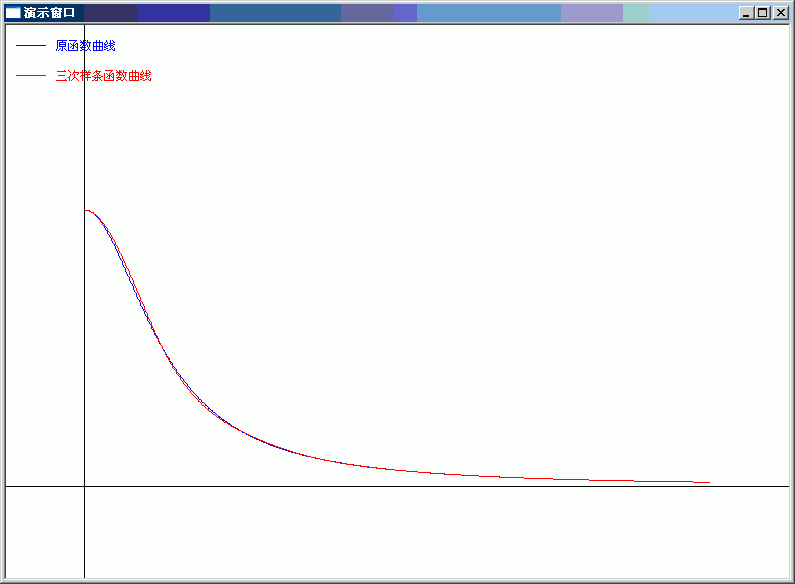图12－2 拟合曲线和原始曲线对比

从图12－2可以看到，三次样条曲线拟合的效果非常好。同样在[0.0, 8.0]区间上，如果增加插值点的个数，将获得更好的拟合效果。比如以0.5为单位，将插值点增加到17个，则拟合的曲线与原始曲线几乎完全重合。


展开全文算法 c++ 数学计算 技术
• ## 曲线拟合

万次阅读 2015-05-01 00:40:48
曲线拟合(Curve Fitting)的数学定义是指用连续曲线近似地刻画或比拟平面上一离散点所表示的坐标之间的函数关系，是一种用解析表达式逼近离散数据的方法。曲线拟合通俗的说法就是“拉曲线”，也就是将现有数据...

12.1 曲线拟合

12.1.1 曲线拟合的定义

曲线拟合(Curve Fitting)的数学定义是指用连续曲线近似地刻画或比拟平面上一组离散点所表示的坐标之间的函数关系，是一种用解析表达式逼近离散数据的方法。曲线拟合通俗的说法就是“拉曲线”，也就是将现有数据透过数学方法来代入一条数学方程式的表示方法。科学和工程遇到的很多问题，往往只能通过诸如采样、实验等方法获得若干离散的数据，根据这些数据，如果能够找到一个连续的函数（也就是曲线）或者更加密集的离散方程，使得实验数据与方程的曲线能够在最大程度上近似吻合，就可以根据曲线方程对数据进行数学计算，对实验结果进行理论分析，甚至对某些不具备测量条件的位置的结果进行估算。

12.1.2 简单线性数据拟合的例子

回想一下中学物理课的“速度与加速度”实验：假设某物体正在做加速运动，加速度未知，某实验人员从时间t0 = 3秒时刻开始，以1秒时间间隔对这个物体连续进行了12次测速，得到一组速度和时间的离散数据，请根据实验结果推算该物体的加速度。

时间 (秒)

3

4

5

6

7

8

9

10

速度（米/秒）

8.41

9.94

11.58

13.02

14.33

15.92

17.54

19.22

时间 (秒)

11

12

13

14

速度（米/秒）

20.49

22.01

23.53

24.47

表 12 – 1 物体速度和时间的测量关系表

在选择了合适的坐标刻度之后，我们就可以在坐标纸上画出这些点。如图12–1所示，排除偏差明显偏大的测量值后，可以看出测量结果呈现典型的线性特征。沿着该线性特征画一条直线，使尽量多的测量点能够位于直线上，或与直线的偏差尽量小，这条直线就是我们根据测量结果拟合的速度与时间的函数关系。最后在坐标纸上测量出直线的斜率K，K就是被测物体的加速度，经过测量，我们实验测到的物体加速度值是1.48米/秒2。

图 12 – 1 实验法测量加速度的过程

12.2 最小二乘法曲线拟合

使用数学分析进行曲线拟合有很多常用的方法，这一节我们先介绍一下最简单的最小二乘法，并给出使用最小二乘法解决上一节给出的速度与加速度实验问题。

12.2.1 最小二乘法原理

下面以多项式模式为例，介绍一下使用最小二乘法进行曲线拟合的完整步骤。假设选择的拟合多项式模式是：这m个等式相当于m个方程，a0,a1,…am是m个未知量，因此这m个方程组成的方程组是可解的，最小二乘法的第二步处理就是将其整理为针对a0,a1,…am的正规方程组。最终整理的方程组如下：最小二乘法的第三步处理就是求解这个多元一次方程组，得到多项式的系数a0,a1,…am，，就可以得到曲线的拟合多项式函数。求解多元一次方程组的方法很多，高斯消元法是最常用的一种方法，下一节就简单介绍一下最小二乘算法实现所用的高斯消元法算法。

12.2.2 高斯消元法求解方程组

在数学上，高斯消元法是线性代数中的一个算法，可用来求解多元一次线性方程组，也可以用来求矩阵的秩，以及求可逆方阵的逆矩阵。高斯消元法虽然以数学家高斯的名字命名，但是最早出现在文献资料中应该是中国的《九章算术》。

高斯消元法的主要思想是通过对系数矩阵进行行变换，将方程组的系数矩阵由对称矩阵变为三角矩阵，从而达到消元的目的，最后通过回代逐个获得方程组的解。在消元的过程中，如果某一行的对角线元素的值太小，在计算过程中就会出现很大的数除以很小的数的情况，有除法溢出的可能，因此在消元的过程中，通常都会增加一个主元选择的步骤，通过行交换操作，将当前列绝对值最大的行交换到当前行位置，避免了除法溢出问题，增加了算法的稳定性。

高斯消元法算法实现简单，主要有两个步骤组成，第一个步骤就是通过选择主元，逐行消元，最终行程方程组系数矩阵的三角矩阵形式，第二个步骤就是逐步回代的过程，最终矩阵的对角线上的元素就是方程组的解。下面就给出高斯消元法的一个算法实现：

76 /*带列主元的高斯消去法解方程组，最后的解在matrixA的对角线上*/
77 bool GuassEquation::Resolve(std::vector<double>& xValue)
78 {
79     assert(xValue.size() == m_DIM);
80
81     /*消元，得到上三角阵*/
82     for(int i = 0; i < m_DIM - 1; i++)
83     {
84         /*按列选主元*/
85         int pivotRow = SelectPivotalElement(i);
86         if(pivotRow != i)/*如果有必要，交换行*/
87         {
88             SwapRow(i, pivotRow);
89         }
90         if(IsPrecisionZero(m_matrixA[i * m_DIM + i])) /*主元是0?
不存在唯一解*/
91         {
92             return false;
93         }
94         /*对系数归一化处理，使行第一个系数是1.0*/
95         SimplePivotalRow(i, i);
96         /*逐行进行消元*/
97         for(int j = i + 1; j < m_DIM; j++)
98         {
99             RowElimination(i, j, i);
100         }
101     }
102     /*回代求解*/
103     m_matrixA[(m_DIM - 1) * m_DIM + m_DIM - 1] = m_bVal[m_DIM - 1] /m_matrixA[(m_DIM - 1) * m_DIM + m_DIM - 1];
104     for(int i = m_DIM - 2; i >= 0; i--)
105     {
106         double totalCof = 0.0;
107         for(int j = i + 1; j < m_DIM; j++)
108         {
109             totalCof += m_matrixA[i * m_DIM + j] * m_matrixA[j * m_DIM + j];
110         }
111         m_matrixA[i * m_DIM + i] = (m_bVal[i] - totalCof) / m_matrixA[i * m_DIM+ i];
112     }
113
114     /*将对角线元素的解逐个存入解向量*/
115     for(int i = 0; i < m_DIM; i++)
116     {
117         xValue[i] = m_matrixA[i * m_DIM + i];
118     }
119
120     return true;
121 }

GuassEquation::Resolve()函数中m_matrixA是以一维数组形式存放的系数矩阵，m_DIM是矩阵的维数，SelectPivotalElement()函数从系数矩阵的第i列中选择绝对值最大的那个值所在的行，并返回行号，SwapRow()函数负责交换系数矩阵两个行的所有值，SimplePivotalRow()函数是归一化处理函数，通过除法操作将指定的行的对角线元素变换为1.0，以便简化随后的消元操作。

12.2.3 最小二乘法解决“速度与加速度”实验

根据12.2.1节对最小二乘法原理的分析，用程序实现最小二乘法曲线拟合的算法主要由两个步骤组成，第一个步骤就是根据给出的测量值生成关于拟合多项式系数的方程组，第二个步骤就是解这个方程组，求出拟合多项式的各个系数。根据对上文最终整理的正规方程组的分析，可以看出其系数有一定的关系，就是每一个方程式都比前一个方程式多乘了一个xi。因此，只需要完整计算出第一个方程式的系数，其他方程式的系数只是将前一个方程式的系数依次左移一位，然后单独计算出最后一个系数就可以了，此方法可以减少很多无谓的计算。求解多元一次方程组的方法就使用12.2.2节介绍的高斯消元法，其算法上一节已经给出。

这里给出一个最小二乘算法的完整实现，以12.1.2节的数据为例，因为数据结果明显呈现线性方程的特征，因此选择拟合多项式为v = v0 + at，v0和a就是要求解的拟合多项式系数。

99 bool LeastSquare(const std::vector<double>& x_value, const std::vector<double>&y_value,
100                  int M, std::vector<double>& a_value)
101 {
102     assert(x_value.size() == y_value.size());
103     assert(a_value.size() == M);
104
105     double *matrix = new double[M * M];
106     double *b= new double[M];
107
108     std::vector<double> x_m(x_value.size(), 1.0);
109     std::vector<double> y_i(y_value.size(), 0.0);
110     for(int i = 0; i < M; i++)
111     {
112         matrix[ARR_INDEX(0, i, M)] = std::accumulate(x_m.begin(), x_m.end(),0.0);
113         for(int j = 0; j < static_cast<int>(y_value.size()); j++)
114         {
115             y_i[j] = x_m[j] * y_value[j];
116         }
117         b[i] = std::accumulate(y_i.begin(), y_i.end(), 0.0);
118         for(int k = 0; k < static_cast<int>(x_m.size()); k++)
119         {
120             x_m[k] *= x_value[k];
121         }
122     }
123     for(int row = 1; row < M; row++)
124     {
125         for(int i = 0; i < M - 1; i++)
126         {
127             matrix[ARR_INDEX(row, i, M)] = matrix[ARR_INDEX(row - 1, i + 1, M)];
128         }
129         matrix[ARR_INDEX(row, M - 1, M)] = std::accumulate(x_m.begin(),x_m.end(), 0.0);
130         for(int k = 0; k < static_cast<int>(x_m.size()); k++)
131         {
132             x_m[k] *= x_value[k];
133         }
134     }
135
136     GuassEquation equation(M, matrix, b);
137     delete[] matrix;
138     delete[] b;
139
140     return equation.Resolve(a_value);
141 }

将表12－1的数据带入算法，计算得到v0 = 4.05545455，a = 1.48818182，比作图法得到的结果更精确。以上算法是根据最小二乘法的理论推导系数方程，并求解系数方程得到拟合多项式的系数的一种实现方法，除此之外，还可以利用预先计算好的最小二乘解析理论直接求得拟合多项式的系数，读者可自行学习相关的实现算法。

12.3 三次样条曲线拟合

Basis Function）的曲线拟合方法需要高深的数学基础，涉及多维空间理论，将低维的模式输入数据转换到高维空间中，使得低维空间内的线性不可分问题在高维空间内变得线性可分，这种数学分析方法非常强大，但是这种方法不宜得到拟合函数，因此在需要求解拟合函数的情况下使用起来不是很方便。

样条插值是一种工业设计中常用的、得到平滑曲线的一种插值方法，三次样条又是其中用的较为广泛的一种。使用三次样条曲线进行曲线拟合可以得到非常高精度的拟合结果，并且很容易得到拟合函数，本节的内容将重点介绍三次样条曲线拟合的原理和算法实现，并通过一个具体的例子将三次样条函数拟合的曲线与原始曲线对比显示，体会一下三次样条曲线拟合的惊人效果。

12.3.1 插值函数

前文提到过，曲线拟合的实质就是各种插值计算，因此，插值函数的选择决定了曲线拟合的效果。那么插值函数的数学定义是什么呢？若在[a, b]上给出n + 1个点a ≤ x0 < x1 < ⋯ < xn ≤ b，f(x) 是[a, b]上的实值函数, 要求一个具有n + 1个参量的函数s(x; a0,...,an) 使它满足

s(xi; a0,..., an) = f(xi) , i = 0, 1, ⋯, n                          （3.5）

则称s(x)为f(x) 在[a, b]上的插值函数. 若s(x) 关于参量a0, a1,...,an是线性关系, 即：

s(x) = a0s0(x) + a1s1(x) + ⋯ + ansn(x)                                （3.6）

s(x)就是多项式插值函数，如果si(x)是三角函数，则s(x)就是三角插值函数。

比较常用的多项式插值函数是牛顿插值多项式和拉格朗日插值多项式，但是在多项式的次数比较高的情况下，插值点数n过多会导致多项式插值在收敛性和稳定性上失去保证，因此，当插值点数n较大的情况下，一般不使用多项式插值，而采用样条插值或次数较低的最小二乘法插值。

12.3.2 样条函数的定义

在所有能够保证收敛性和稳定性的插值函数中，最常用的，也是最重要的插值函数就是样条插值函数。采用样条函数计算出的插值曲线和曲面在飞机、轮船和汽车等精密机械设计中都得到了广泛的应用。样条插值函数的数学定义是这样的：

设区间[a, b]上选取n - 1个节点（包括区间端点a和b共n + 1个节点），将其划分为n个子区间 a = x0 < x1 < ⋯ < xn = b, 如果存在函数s(x)，使得s(x)满足以下两个条件：

(1) s(x) 在整个区间[a, b]上具有m - 1阶连续导数；

(2) s(x)在每个子区间[xi-1, xi], i= 1, 2, ⋯, n 上是m 次代数多项式（最高次数为m次）；

则称s(x)是区间[a, b]上的m 次样条函数。假如区间[a, b]上存在实值函数f(x)，使得每个节点处的值f(xi)与s(xi)相等，即

s(xi) = f(xi), i = 0, 1, …, n                                          （3.7）

则称s(x)是实值函数f(x)的m 次样条插值函数。

当m = 1时，样条插值函数就是分段线性插值, 此时虽然s(x)是属于区间[a, b]上的函数, 但它不光滑（连一阶连续导数性质都不具备）,不能满足工程设计要求。工程设计通常使用较多的是m = 3时的三次样条插值函数，此时样条函数具有二阶连续导数性质。

根据三次样条函数的定义，s(x)在每个子区间上的样条函数si(x)都是一个三次多项式，也就是说，三次样条函数s(x)由n个区间上的n个三次多项式组成，每个三次多项式可描述为以下形式：

si(x) = aix3 + bix2 + cix + di      i = 1, 2, …, n                      （3.8）

因此，要确定完整的样条函数s(x)需要确定ai、bi、ci和di公4n个系数。根据样条函数的定义，s(x)在区间内的n - 1个节点处都是连续的，并且其一阶导数si‘(x)和二阶导数si“(x)都是连续的，根据连续函数的性质（xi的左右导数相等），我们可以得到3(n - 1)个条件：

si(xi - 0) = si+1(xi + 0)    i = 1, 2, …, n-1

si‘(xi - 0) = si+1‘(xi + 0)    i = 1, 2, …, n-1

si“(xi - 0) = si+1“(xi + 0)    i = 1, 2, …, n-1                          （3.9）

再加上插值函数在包括区间端点a（就是x0），b（就是xn）在内的n + 1个节点处满足s(xi) = f(xi)，又可以得到n + 1个条件，这样就具备了4n – 2个条件。

12.3.3 边界条件

为了解决4n个系数组成的方程组，最终确定的s(x)，需要再补充两个边界条件使之满足4n个条件。常用的边界条件有以下几种：

第一类边界条件，即满足s‘(x0) = f’(x0)，s‘(xn) = f’(xn)两个条件，其中f(x)是实值函数。

第二类边界条件，即满足s”(x0) = f”(x0)，s”(xn) = f”(xn)两个条件，其中f(x)是实值函数。特别情况下，当f”(x0) = f”(xn) = 0的时候，也就是s”(x0) = s”(xn) = 0的情况下，第二类边界条件又被称为自然边界条件。

当样条函数的实值函数f(x)是以[a, b]为周期的周期函数时，三次样条函数s(x)在两个端点处满足s‘(x0 - 0) = s‘(xn + 0)和s”(x0 - 0) = s”(xn + 0)，这种情况又被成为第三类边界条件。

工程技术中常用的是第一类边界条件和第二类边界条件，以及第二类边界条件的特殊情况自然边界条件。理想情况下，也就是实值函数已知的情况下，可以通过实值函数直接计算出边界条件的值，否则的话，就只能通过测量和计算得到边界条件的值，有时候甚至只能给出经验估计值，工程技术中通常根据实际情况灵活使用各类边界条件。

12.3.4 推导三次样条函数

求三次样条插值函数s(x)的方法很多，其基本原理都是首先求出由待定系数组成的s(x)，以及其一阶导数s’(x)和二阶导数s”(x)，然后将其带入到12.3.2和12.3.3节列举的4n个条件中，得到关于待定系数的方程组，最后求解方程组得到待定系数，并最终确定插值函数s(x)。

求三次样条插值函数s(x)常用的方法是“三转角法”和“三弯矩法”。根据三次样条函数的性质，s(x)的一阶导数s’(x)是二次多项式， 二阶导数s”(x)是一次多项式（线性函数）， “三转角法”和“三弯矩法”的主要区别是利用这两个特性推导插值函数s(x)、s’(x)和s”(x)的方式不同。“三转角法”利用s(x)的一阶导数s’(x)是二次多项式这个特性，对于子区间[xi, xi+1]，利用抛物线插值公式获得一个通过xi和xi+1两个点的二次多项式做为s’(x)，然后对s’(x)进行积分和微分（求导）运算，分别得到s(x)，和s”(x)，最后将它们带入4n个条件中求解系数方程组。“三弯矩法”则是利用s(x)的二阶导数s”(x)是一次多项式（线性函数）这个特性，对于子区间[xi,
xi+1]，首先假设一个通过xi和xi+1两个点的线性函数做为s”(x)，然后对s”(x)进行连续两次积分运算得到s(x)，再对s(x)进行求导得运算到s’(x)，最后将它们带入4n个条件中求解系数方程组。这两种方法的本质是一样的，只是对s(x)的推导过程不同，接下来就介绍使用“三弯矩法”求解三次样条函数的方法。

三次样条函数的求解过程就是系数方程组的推导过程，使用“三弯矩法”推导系数方程组，首先要确定插值函数的二阶导数s”(x)。根据三次样条函数的性质，在每个子区间[xi, xi+1]上，其二阶导数s”(x)是个线性方程，现在假设在xi和xi+1两个端点的二阶导数值分别是Mi和Mi+1，也就是s”(xi) = Mi，s”(xi+1)
= Mi+1，则经过xi和xi+1的两点式直线方程是：从M0到Mn，有n+1个Mi的值需要求解，但是（3.20）只有n-1个等式，此时就需要用到两个边界条件了。

如果是使用第二类边界条件，则直接可以得到以下两个条件等式：

s”(x0) = M0 = f”(x0) = y0’                                                (3.21)

s”(xn) = Mn = f”(xn) = yn’                                                (3.22)

令d0 = 2y0’，dn = 2yn’，可以得到由第二类边界条件确定的两个方程：

2M0 = d0                                                                    (3.23)

2Mn = dn                                                                    (3.24)

如果是使用第一类边界条件，即s‘(x0) = f’(x0)，s‘(xn) = f’(xn)两个条件，则需要将这两个条件代入（3.16），通过计算得到两个条件等式。将s‘(x0) = y0’代入（3.16），得到：将第二类边界条件得到的(3.23)和（3.24）或第一类边界条件得到的（3.27）和（3.28）与（3.20）中的n – 1个等式组合在一起就得到一个关于Mi的方程组，求解此方程组可以得到Mi的值，代入到（3.15）即可得到三次样条函数方程。以第一类边界条件得到的（3.27）和（3.28）为例，与（3.20）连立得到以下方程组：这就是三弯矩方程组，其中Mi，i=0,1,…n就是三次样条函数s(x)的矩。根据（3.27）和（3.28），un = 1，v0 = 1，其余各系数可以通过（3.19）中的系数计算出来。这个方程组的系数矩阵是一个对角线矩阵，并且是一个严格对角占优的对角阵（ui和vi的值均小于主对角线的值，也就是ui和vi的值皆小于2），可以使用追赶法求解。下一节将介绍如何使用追赶法求解方程组，并给出求解的算法实现。

12.3.5 追赶法求解方程组

任意矩阵A，都可以通过克洛脱(Crout)分解得到两个三角矩阵：其中y1=d1/l1，其余各项的递推计算关系是：

yi = (di – miyi-1)/li， i = 2，3，… ，n

对于第二个方程，求解最终结果xi：其中xn = yn，其余各项的递推求解关系是：

xi = yi – uixi+1， i = n – 1, n – 2, …,
1

递推计算yi和xi的过程分别被形象地形容为追的过程和赶的过程，这也是追赶法得名的原因，实际上这种方法在国际上叫做托马斯（Thomas）法。在这里需要强调一下，对三角矩阵的克洛脱分解需要满足几个条件，否则无法进行，这几个条件分别是：下面就给出一个追赶法求解方程组的通用算法实现，在使用之前需要判断系数矩阵是否是对三角矩阵，并且满足上述三个条件，相关的判断请读者自行添加：

76 /*追赶法求对三角矩阵方程组的解*/
77 bool ThomasEquation::Resolve(std::vector<double>& xValue)
78 {
79     assert(xValue.size() == m_DIM);
80
81     std::vector<double> L(m_DIM);
82     std::vector<double> M(m_DIM);
83     std::vector<double> U(m_DIM);
84     std::vector<double> Y(m_DIM);
85
86     /*消元，追的过程*/
87     L = m_matrixA[ARR_INDEX(0, 0, m_DIM)];
88     U = m_matrixA[ARR_INDEX(0, 1, m_DIM)] / L;
89     Y = m_bVal / L;
90     for(int i = 1; i < m_DIM; i++)
91     {
92         if(IsPrecisionZero(m_matrixA[ARR_INDEX(i, i, m_DIM)]))
93         {
94             return false;
95         }
96         M[i] = m_matrixA[ARR_INDEX(i, i - 1, m_DIM)];
97         L[i] = m_matrixA[ARR_INDEX(i, i, m_DIM)] - M[i] * U[i - 1];
98         Y[i] = (m_bVal[i] - M[i] * Y[i - 1]) / L[i];
99         if((i + 1) < m_DIM)
100         {
101             U[i] = m_matrixA[ARR_INDEX(i, i + 1, m_DIM)] / L[i];
102         }
103     }
104     /*回代求解，赶的过程*/
105     xValue[m_DIM - 1] = Y[m_DIM - 1];
106     for(int i = m_DIM - 2; i >= 0; i--)
107     {
108         xValue[i] = Y[i] - U[i] * xValue[i + 1];
109     }
110
111     return true;
112 }

12.3.6 三次样条曲线拟合算法实现

根据12.3.4节对三次样条函数的推导分析，三次样条曲线拟合算法的核心可分为三部分，第一部分是根据推导结果计算关于三次样条函数的“矩”的方程组的系数矩阵，第二部分就是用追赶法求解方程组，得到各个区间的三次样条函数，第三部分就是根据每个拟合点的输入的值xi，确定使用哪个区间的三次样条函数，并使用该区间的三次样条函数计算出三次样条插值yi，最后得到的一系列（xi,
yi）组成的曲线就是三次样条拟合曲线。拟合算法也是按照上面的分析，分三个步骤计算插值：

第一步是计算系数矩阵，其中u0、v0、d0和dn的值需要单独计算，其余的值可以通过（3.19）递推计算出来。

第二步是将系数矩阵代入12.3.5节给出的追赶法通用算法，求出Mi的值。求解之前，先证明一下第一步得到系数矩阵是否满足追赶法的条件。首先，主对角线元素的值都是2，满足12.3.5节的条件（1）。其次，由ui和vi的计算条件可知，|ui| < 1，|vi| < 1，这也满足12.3.5节的条件（2）。最后，因为ai = 2，且ui和vi的和是1，所以12.3.5节的条件（3）也得到了满足。由上判断可知，求解三次样条函数的“矩”的系数矩阵满足使用追赶法求解的条件，可以使用追赶法求解。

第三步是计算插值，需要将第二步计算得到的Mi代入（3.15），并选择合适的子区间样条函数计算出插值点的值。

下面就给出采用三弯矩法实现的三次样条曲线拟合算法，CalcSpline()函数的参数Xi和Yi是n个插值点（包括起点和终点）的值，boundType是边界条件类型，b1和b2分别是对应的两个边界条件，这个算法支持第一类和第二类边界条件（包括自然边界条件）。内部的矩阵matrixA就是按照（3.29）构造的Mi方程组的系数矩阵，可用于直接用追赶法求解方程组。CalcSpline()函数的大部分代码都是在构造Mi方程组的系数矩阵，首先根据边界条件确定un 、v0 、d0和dn，其他系数则根据（3.19）的递推关系，在for(int
i = 1; i < (m_valN - 1); i++)循环中依次计算出来，最后是利用12.3.5节给出的追赶法算法求出Mi。GetValue()函数负责计算给定区间内任意位置的插值，首先根据x的值确定使用哪个子区间的样条函数，然后根据（3.12）和（3.14）给出的关系计算插值。

16 void SplineFitting::CalcSpline(double *Xi, double *Yi, int n, int boundType,double b1, double b2)
17 {
18     assert((boundType == 1) || (boundType == 2));
19
20     double *matrixA = new double[n * n];
21     if(matrixA == NULL)
22     {
23         return;
24     }
25     double *d = new double[n];
26     if(d == NULL)
27     {
28         delete[] matrixA;
29         return;
30     }
31
32     m_valN = n;
33     m_valXi.assign(Xi, Xi + m_valN);
34     m_valYi.assign(Yi, Yi + m_valN);
35     m_valMi.resize(m_valN);
36     memset(matrixA, 0, sizeof(double) * n * n);
37
38     matrixA[ARR_INDEX(0, 0, m_valN)] = 2.0;
39     matrixA[ARR_INDEX(m_valN - 1, m_valN - 1, m_valN)] = 2.0;
40     if(boundType == 1) /*第一类边界条件*/
41     {
42         matrixA[ARR_INDEX(0, 1, m_valN)] = 1.0; //v0
43         matrixA[ARR_INDEX(m_valN - 1, m_valN - 2, m_valN)] = 1.0; //un
44         double h0 = Xi - Xi;
45         d = 6 * ((Yi - Yi) / h0 - b1) / h0; //d0
46         double hn_1 = Xi[m_valN - 1] - Xi[m_valN - 2];
47         d[m_valN - 1] = 6 * (b2 - (Yi[m_valN - 1] - Yi[m_valN - 2]) / hn_1) /hn_1; //dn
48     }
49     else /*第二类边界条件*/
50     {
51         matrixA[ARR_INDEX(0, 1, m_valN)] = 0.0; //v0
52         matrixA[ARR_INDEX(m_valN - 1, m_valN - 2, m_valN)] = 0.0; //un
53         d = 2 * b1; //d0
54         d[m_valN - 1] = 2 * b2; //dn
55     }
56     /*计算ui,vi,di，i = 2,3,...,n-1*/
57     for(int i = 1; i < (m_valN - 1); i++)
58     {
59         double hi_1 = Xi[i] - Xi[i - 1];
60         double hi = Xi[i + 1] - Xi[i];
61         matrixA[ARR_INDEX(i, i - 1, m_valN)] = hi_1 / (hi_1 + hi); //ui
62         matrixA[ARR_INDEX(i, i, m_valN)] = 2.0;
63         matrixA[ARR_INDEX(i, i + 1, m_valN)] = 1 - matrixA[ARR_INDEX(i, i - 1,m_valN)]; //vi
= 1 - ui
64         d[i] = 6 * ((Yi[i + 1] - Yi[i]) / hi - (Yi[i] - Yi[i - 1]) / hi_1) /(hi_1 + hi); //di
65     }
66
67     ThomasEquation equation(m_valN, matrixA, d);
68     equation.Resolve(m_valMi);
69     m_bCalcCompleted = true;
70
71     delete[] matrixA;
72     delete[] d;
73 }
74
75 double SplineFitting::GetValue(double x)
76 {
77     if(!m_bCalcCompleted)
78     {
79         return 0.0;
80     }
81     if((x < m_valXi) || (x > m_valXi[m_valN - 1]))
82     {
83         return 0.0;
84     }
85     int i = 0;
86     for(i = 0; i < (m_valN - 1); i++)
87     {
88         if((x >= m_valXi[i]) && (x < m_valXi[i + 1]))
89             break;
90     }
91     double hi = m_valXi[i + 1] - m_valXi[i];
92     double xi_1 = m_valXi[i + 1] - x;
93     double xi = x - m_valXi[i];
94
95     double y = xi_1 * xi_1 * xi_1 * m_valMi[i] / (6 * hi);
96     y += (xi * xi * xi * m_valMi[i + 1] / (6 * hi));
97
98     double Ai = (m_valYi[i + 1] - m_valYi[i]) / hi - (m_valMi[i + 1] -m_valMi[i]) * hi / 6.0;
99     y += Ai * x;
100     double Bi = m_valYi[i + 1] - m_valMi[i + 1] * hi * hi / 6.0 - Ai * m_valXi[i+ 1];
101     y += Bi;
102     return y;
103 }

12.3.7 三次样条曲线拟合的效果

本节将用定义一个原始函数，从原始函数的某个区间上抽取9个插值点，根据这9个插值点和原函数的边界条件，利用三次样条曲线插值进行曲线拟合，并将原始曲线和拟合曲线做对比，展示一下三次样条曲线拟合的效果。

首先定义原始函数：

f(x) = 3/(1 + x2)

选择区间[0.0, 8.0]上的9个点做为插值点，计算各点的值为：

x

0.0

1.0

2.0

3.0

4.0

5.0

6.0

7.0

8.0

y

3.0

1.5

0.6

0.3

0.1765

0.1154

0.0811

0.06

0.0462

表 12 – 2 原函数f(x)在各插值点的值

求f(x)的导函数f’(x)：

f’(x) = -6x/(1 + x2)2

根据f’(x)计算出在区间端点处的两个第一类边界条件f’(0.0) = 0.0, f’(8.0) = -0.01136。利用表12－2中的数据和这两个边界条件，计算出三次样条插值函数，并从0.0开始，以0.01为步长，连续求800个点的插值，将这些点连成曲线得到拟合曲线。为了作对比，同样从0.0开始，以0.01为步长，用f(x)函数连续计算800个点的原值，将这些点连成曲线得到原始曲线。分别用不同的颜色画出这两条曲线，如图12－2所示：图12－2 拟合曲线和原始曲线对比

从图12－2可以看到，三次样条曲线拟合的效果非常好。同样在[0.0, 8.0]区间上，如果增加插值点的个数，将获得更好的拟合效果。比如以0.5为单位，将插值点增加到17个，则拟合的曲线与原始曲线几乎完全重合。

展开全文算法
• 最近在弄一些数据趋势判断该方面的东西，下面代码是网友使用最小二乘法做的数据拟合算法的java实现 public class PolyFitForcast { public PolyFitForcast() { } /** * * 函数功能：最小二乘法曲线拟合 ...
最近在弄一些数据趋势判断该方面的东西，下面代码是网友使用最小二乘法做的数据拟合算法的java实现
public class PolyFitForcast {
public PolyFitForcast() {
}

/**
* <p>
* 函数功能：最小二乘法曲线拟合
* </p>
* <p>
* 方程:Y = a(0) + a(1) * (X - X1)+ a(2) * (X - X1)^2 + ..... .+ a(m) * (X -
* X1)^m X1为X的平均数
* </p>
*
* @param x
*            实型一维数组,长度为 n. 存放给定 n 个数据点的 X 坐标
* @param y
*            实型一维数组,长度为 n.存放给定 n 个数据点的 Y 坐标
* @param n
*            变量。给定数据点的个数
* @param a
*            实型一维数组，长度为 m.返回 m-1 次拟合多项式的 m 个系数
* @param m
*            拟合多项式的项数，即拟合多项式的最高次数为 m-1. 要求 m<=n 且m<=20。若 m>n 或 m>20
*            ，则本函数自动按 m=min{n,20} 处理.
*            <p>
*            Date:2007-12-25 16:21 PM
*            </p>
* @author qingbao-gao
* @return 多项式系数存储数组
*/
public static double[] PolyFit(double x[], double y[], int n, double a[],
int m) {
int i, j, k;
double z, p, c, g, q = 0, d1, d2;
double[] s = new double;
double[] t = new double;
double[] b = new double;
double[] dt = new double;
for (i = 0; i <= m - 1; i++) {
a[i] = 0.0;
}
if (m > n) {
m = n;
}
if (m > 20) {
m = 20;
}
z = 0.0;
for (i = 0; i <= n - 1; i++) {
z = z + x[i] / (1.0 * n);
}
b = 1.0;
d1 = 1.0 * n;
p = 0.0;
c = 0.0;
for (i = 0; i <= n - 1; i++) {
p = p + (x[i] - z);
c = c + y[i];
}
c = c / d1;
p = p / d1;
a = c * b;
if (m > 1) {
t = 1.0;
t = -p;
d2 = 0.0;
c = 0.0;
g = 0.0;
for (i = 0; i <= n - 1; i++) {
q = x[i] - z - p;
d2 = d2 + q * q;
c = c + y[i] * q;
g = g + (x[i] - z) * q * q;
}
c = c / d2;
p = g / d2;
q = d2 / d1;
d1 = d2;
a = c * t;
a = c * t + a;
}
for (j = 2; j <= m - 1; j++) {
s[j] = t[j - 1];
s[j - 1] = -p * t[j - 1] + t[j - 2];
if (j >= 3)
for (k = j - 2; k >= 1; k--) {
s[k] = -p * t[k] + t[k - 1] - q * b[k];
}
s = -p * t - q * b;
d2 = 0.0;
c = 0.0;
g = 0.0;
for (i = 0; i <= n - 1; i++) {
q = s[j];
for (k = j - 1; k >= 0; k--) {
q = q * (x[i] - z) + s[k];
}
d2 = d2 + q * q;
c = c + y[i] * q;
g = g + (x[i] - z) * q * q;
}
c = c / d2;
p = g / d2;
q = d2 / d1;
d1 = d2;
a[j] = c * s[j];
t[j] = s[j];
for (k = j - 1; k >= 0; k--) {
a[k] = c * s[k] + a[k];
b[k] = t[k];
t[k] = s[k];
}
}
dt = 0.0;
dt = 0.0;
dt = 0.0;
for (i = 0; i <= n - 1; i++) {
q = a[m - 1];
for (k = m - 2; k >= 0; k--) {
q = a[k] + q * (x[i] - z);
}
p = q - y[i];
if (Math.abs(p) > dt) {
dt = Math.abs(p);
}
dt = dt + p * p;
dt = dt + Math.abs(p);
}
return a;
}// end

/**
* <p>
* 对X轴数据节点球平均值
* </p>
*
* @param x
*            存储X轴节点的数组
*            <p>
*            Date:2007-12-25 20:21 PM
*            </p>
* @author qingbao-gao
* @return 平均值
*/
public static double average(double[] x) {
double ave = 0;
double sum = 0;
if (x != null) {
for (int i = 0; i < x.length; i++) {
sum += x[i];
}
ave = sum / x.length;
}
return ave;
}

/**
* <p>
* 由X值获得Y值
* </p>
* @param x
*            当前X轴输入值,即为预测的月份
* @param xx
*            当前X轴输入值的前X数据点
* @param a
*            存储多项式系数的数组
* @param m
*            存储多项式的最高次数的数组
*            <p>
*            Date:2007-12-25 PM 20:07
*            </p>
* @return 对应X轴节点值的Y轴值
*/
public static double getY(double x, double[] xx, double[] a, int m) {
double y = 0;
double ave = average(xx);

double l = 0;
for (int i = 0; i < m; i++) {
l = a;
if (i > 0) {
y += a[i] * Math.pow((x - ave), i);
}
}
return (y + l);
}

public static void main(String[] args) throws Exception{

double[] test_xx = { 0, 1, 2, 3, 4, 5, 6, 7, 8, 9, 10,
11, 12, 13,14,15,16,17,18,19,20,21,22,23,24,25,26,27,28,29,30,
31,32,33,34,35,36,37,38,39,40,
41,42,43,44,45,46,47,48,49,50,
51,52,53,54,55,56,57,58,59};// 15
double[] ss  = {
9880,10000,9880,10088,9958,9869,9712,9670,9719,9625,9416,
9238,8962,9034,9375,9555,8162,8589,8119,8112,8092,8105,
7821,7943,8086,7825,7914,7804,7789,7903,7630,7666,
7545,7632,7619,7582,7427,7499,7463,7384,7315,7092,
7123,6911,7024,7016,7009,6929,7093,6972,7008,6689,
6793,6805,6835,6553,6659,6713,6757,6581};

int mmm = ss.length;
int m = 3;
double[] a = new double[test_xx.length];
double[] aa = PolyFit(test_xx, ss, mmm, a, m);
for(int i=0;i<mmm;i++){
System.out.println("拟合-->" + getY(i, test_xx, aa, m));
}
}

}

展开全文• 一种常用的方法，就是对每次实验获得的数据组数据进行近似曲线拟合，从中发现其变化规律。一旦这样的规律可以确定，那么目的就达到了，就可以减少或不必再进行类似的实验了，因为有些实验要重复做是很困难的。对...c
• 如，我们需要从一测定的数据（例如N个点（xi，yi）（i=0,1，…，m））去求得自变量 x 和因变量 y 的一个近似解表达式 y=f（x），这就是由给定的 N 个点（xi，yi）（i=0,1，…，m）求数据拟合的问题。（注意数据...matlab 编程语言
• 通过数据拟合获得二维三维曲线 1.将数据输入到1stOpt中，进行拟合函数的查找，之后呢 2.将得到的函数格式化，运用MATLAB绘画matlab 1stOpt
• ## 车道线拟合笔记

千次阅读 2019-11-07 16:34:06
按作用又可分为：车行道中心线、车道分界线、停止线、减速让行线、人行横道线、导流线、导向箭头和左转弯导线等。 2、目前视觉_车道线检测方法： 一般的车道有三车道或者四车道，固定的前方摄像头的视角范围内，由于...
• ## 二维曲线拟合

千次阅读 2018-11-20 16:49:03
EVD）Householder变换法（QR Factorization,QR分解）奇异值分解（singular value decomposition,SVD）Matlab样条工具箱(Curve Fitting Toolbox)与曲线拟合导入数据，打开工具箱选择合适的模型...
• ## TensorFlow 曲线拟合

万次阅读 2017-09-28 10:58:12
并且当数据超出这些给定的数据范围（x超出-20到20）时，能更好地拟合将来的数据。如图1-1。当数据超出给定训练结果时，如果你计算出的函数正好与原有的曲线函数相对应，则能很好地预测超出的值。 而如果用机器...
• 实验室老师让给数据画一张线性拟合图。不会matlab，就琢磨着用python。参照了网上的一些文章，查看了帮助文档，成功的写了出来 这里用到了三个库 import numpy as np import matplotlib.pyplot as plt from scipy ...Python
• 曲线拟合 最小二乘多项式拟合     寻找一个曲线满足y=f(x)y = f(x)y=f(x)，线性最小二乘法拟合是解决曲线拟合最...式中：rk(x)r_k(x)rk​(x)为实现选定的一线性无关函数；aka_kak​为待定系数
• ## 二次曲线拟合

千次阅读 2014-12-18 00:32:09最小二乘 python
• # -*- coding: cp936 -*- import numpy import pylab def plot_polynomail_fit(matrix,col,*...但是可以对这一组数据同时拟合多条曲线并显示。 matrix:存放的是需要拟合的数据，其中每一列代表一组待拟合数据。 cnumpy
• 例7.2.1 给出一组数据点列入表7–2中，试用线性最小二乘法求拟合曲线，并用（7.2），（7.3）和（7.4）式估计其误差，作出拟合曲线.   表7–2 例7.2.1的一组数据 xi -2.5 -1.7 -1.1 -0.8 0 ...
• 最近在处理b值MRI曲线拟合数据，每数据结构如下 b:[0 20 50 80 100 150 200 400 600 800 1000]; S:[297 283.8 265.2 257.2 256.1 225.8 215.2 169.9 138.5 109.8 101.5];DWI-MRI成像中b值和信号强度的比值...matlab 优化
• ## 曲线拟合与插值

千次阅读 2006-08-08 21:56:00
在大量的应用领域中，人们经常面临用一...人们设法找出某条光滑曲线，它最佳地拟合数据，但不必要经过任何数据点。图11.1说明了这两种方法。标有o的是数据点；连接数据点的实线描绘了线性内插，虚线是数据的最佳拟合matlab plot table equals
• 拟合数据不平衡 什么是过拟合？ 过拟合就是学习器对训练样本数据的学习的过于彻底，将一些训练样本的噪声或者不属于全体样本的一般特征也学习了，造成在训练样本上效果表现很好而在测试样本上表现效果非常...机器学习 正则化
• 3.1 一维插值方法 1、数据处理的应用背景 2、插值的基本原理 3、引例 3.1、引例演示 4、一维插值定义 5、一维插值原理 6、一维插值方法 6.1、拉格朗日插值 6.2、分段线性插值 ...3.3 数据拟合方法 1、拟合的应用背景数学建模 matlab
• 数据拟合和插值方法的汇总介绍，线性回归以及非线性回归
• 曲线拟合 实例：温度曲线问题 气象部门观测到一天某些时刻的温度变化数据为： t 0 1 2 3 4 5 6 7 8 9 10 ...
• 前言：由试验得到一组数据，对该组数据进行处理，作图分析，分析各变量的关系，期望得到拟合公式。 试验数据背景 本次试验有三个自变量：V、M、G，因变量为F,每组试验重复5次，试验目的是探寻F与三个自变量之间的...
• Assembling dataLinearModelFit operates on lists of data. In the easiest case, it uses a list of the form {{Subscript[x, 1],Subscript[y, 1]}, {Subscript[x, 2],Subscript[y, 2]}, ...}....1
• 1.模型的误差产生的机制 • 误差（Error）：模型... 欠拟合：在训练数据和未知数据上表现都很差，高偏差 解决方法： 1）添加其他特征项，有时候我们模型出现欠拟合的时候是因为特征项不够导致的，可以添加其...学习曲线 验证曲线
• 在科学计算和工程应用中，经常会遇到需要拟合一系列的离散数据，最近找了很相关的文章方法，在这里进行总结一下其中最完整、几乎能解决所有参数拟合的方法 第一步：得到散点数据 根据你的实际问题得到一系列的...matlab nlinfit 散点图
• 在上一篇的博客球面数据拟合算法简介中，笔者详细介绍了关于空间球面数据拟合的算法公式推导并给出了相应的Matlab代码及其仿真分析。本次笔者将上面这一情况进行更一般的推广，即取消了球面数据这一限制，数据可以是...算法 matlab
• 首先引入三件套和scipy import numpy as np import pandas as pd import matplotlib.pyplot as plt ...拿到实验数据,通过pandas读取为DataFrame data = pd.read_csv("W-900K.csv") data.head() ......